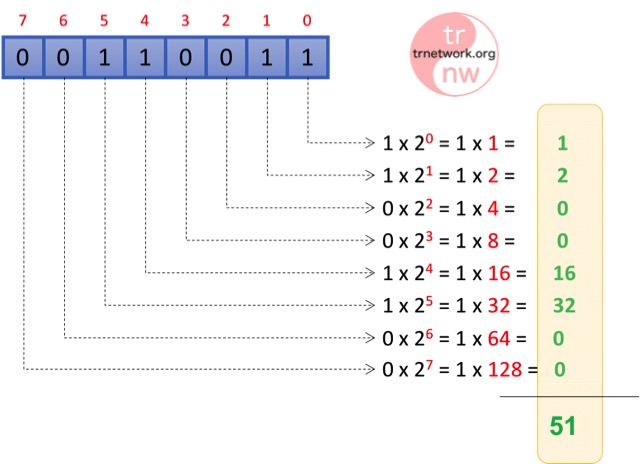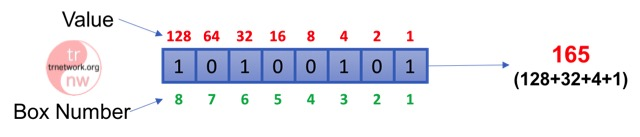# Digital World – Binary and Decimal System

We have to understand the meaning of 0 and 1s which are fundamentals of digital world and electronics for understanding networking basics.

It is hard to believe that, all digital inventions around us are created with using 1 and 0 s, but it is true!

We have an information and we need to transfer this information from one point to another. Despite the fact that the analog systems have been used before, nowadays all information is transferring with digitally. Transferring information with digitally means converting this information to representation of 0 and 1s.

We can say that the first step of digitization begins with Mors alphabet. As you know, at the Mors alphabet, the characters are coding with points and dashes and send over line. At the other side, the other person translates this dots and dashes to the letter.

I will not mention how can be translate data to 0 and 1s here, I want to understand how converted data can be transfer and what the bit and byte terms means.

Every 0 and 1 represents as BIT in digital world. Like as Mors alphabet, one letter represents by combination of dots and dashes, the digital data represents by lot of 0s and 1s. The scientist grouped 8 bits and named is BYTE.

Let’s show a Bit and Byte terms on the figure below;# Converting Binary Number to Decimal

We learned how to convert a binary number to decimal at high school. I am sure you will remember when you see the example below.You remember that? As you see on the figure, if all digits on 1Byte (8bit) will 1, the decimal value represents 255.

So, 255 (mod 10) = 11111111 (mod2)

Let’s we see another example. What is the decimal value of binary value that we give at the first figure 00110011?As you see, the decimal value of 00110011 is 51

Let’s look another aspect,We discard the zeros and only find the decimal equivalent of 1s. If we sum the decimal values, the sum is 51.

According to find decimal value of binary series, we have to know that the decimal equivalent of every bit. You can write down from right box to left box, adding power of 2 respectively.

# Find the Binary Value of Decimal Number

We can find the binary value of decimal number solving numbers reversely.

Let’s make an example. We will find the binary value of 165.Make a box and write all decimal equivalent of digits from right to left.

• Box number 1 decimal eq is = 1
• Box number 2 decimal eq is = 2
• Box number 3 decimal eq is = 4
• Box number 4 decimal eq is = 8
• Box number 5 decimal eq is = 16
• Box number 6 decimal eq is = 32
• Box number 7 decimal eq is = 64
• Box number 8 decimal eq is = 128

• 128 is the nearest box to the 165, so the 8. box has to be 1
• 165-128 = 37 left. 32 is the nearest box to the 37, so 6. Box has to be 1
• 37-32 = 5 left. 4 is the nearest box to the 5, so 3. Box has to be 1.
• 5-4 = 1 left. So, our last box, 1.box has to be 1.

Remember we say before, we only consider 1’s in the bit stream. Other all values will be 0. So, write the green signed boxes 1 and others 0.

Box 8, box 6, box 3 and box 1 are 1, other boxes are 0. Let’s write the bit stream = 10100101You may little confused and asked yourself, what is the relations with this explanations and networking? Yes, it is strongly related with understanding IP addressing, subnet masking, network addressing etc. If we don’t know the conversations between decimal and binary values, we may a problem with understanding some basic network addressing rules. So please make yourself some exercises and clearly understand the conversations.

This site uses Akismet to reduce spam. Learn how your comment data is processed.